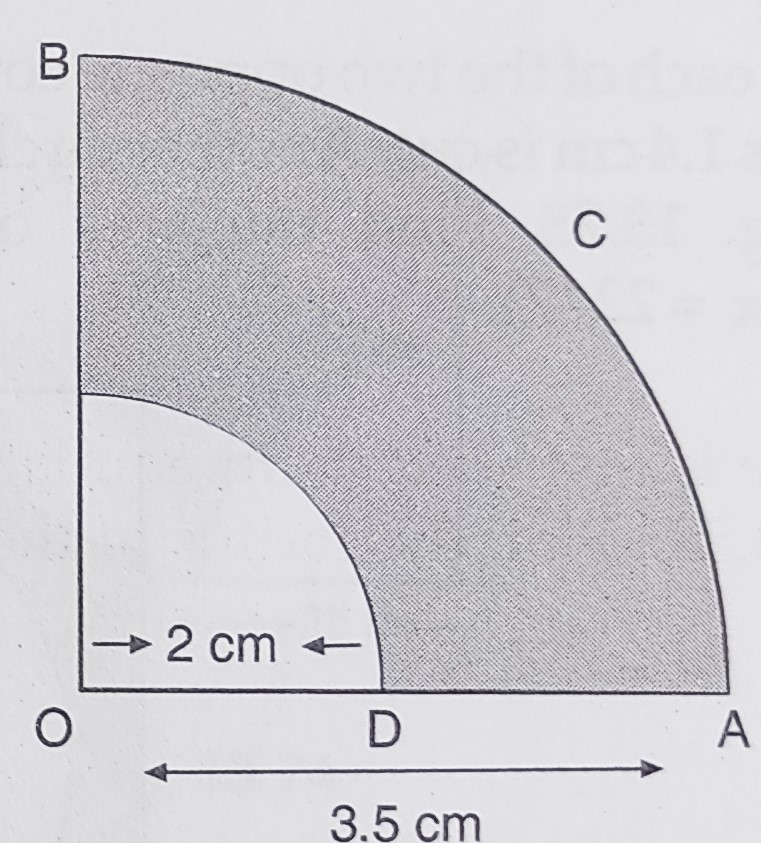"
">

# In the below figure, $O A C B$ is a quadrant of a circle with centre $O$ and radius $3.5 \mathrm{~cm}$. If $O D=2 \mathrm{~cm}$, find the area of the quadrant $O A C B$."

Given:

$O A C B$ is a quadrant of a circle with centre $O$ and radius $3.5 \mathrm{~cm}$.

$O D=2 \mathrm{~cm}$.

To do:

We have to find the area of the quadrant $O A C B$.

Solution:

Radius of the outer quadrant $R = 3.5\ cm$

Radius of the inner quadrant $r= 2\ cm$

This implies,

Area of the quadrant $OACB=\frac{1}{4} \pi \mathrm{R}^{2}$

$=\frac{1}{4} \times \frac{22}{7}(3.5)^{2}$

$=\frac{1}{4} \times \frac{22}{7} \times 3.5 \times 3.5$

$=9.625 \mathrm{~cm}^{2}$

The area of the quadrant $O A C B$ is $9.625\ cm^2$.

Updated on: 10-Oct-2022

40 Views